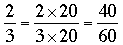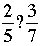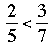SEARCH HOMEMath Central Quandaries & QueriesQuestion from Shea, a teacher: I am a special education teacher, who is trying to to show my students how to order fractions. I found your response to this question while looking for information. I need a visual to explain to my students and myself on how and why you multiply the fractions together. Thanks for your time, SheaHi Shea,

There are two responses on the page you mention, one by Penny and the other by Stephen. I don't know which you are referring to so I will try to explain each of them.

Penny's response:

Penny compares the fractions 2/3, 3/5 and 3/4 using a common denominator. She begins by multiplying the denominators together to get a common denominator. 3 × 5 × 4 = 60. Now consider the fractions one at a time.

2/3: The denominator is 3 and 3 × 20 = 60 so.

Using a similar argument 3/5 = 36/60 and 3/4 = 45/60. Now that the three fractions are expressed with the same denominator you can see that 36/60 < 40/60 < 45/60 so 3/5 < 2/3 < 3/4.

Stephen's response:

Stephen looks at 2/5 and 3/7. He wants to writewhere the question mark is either < or > but he doesn't know which. Regardless of which sign is correct if you multiply both sides by 7 the expression will remain true. Likewise if you multiply both sides by 5 the expression will remain true. Multiplying both sides by 7 and then by 5 gives 2 × 7 ? 3 × 5 or 14 ? 15. Now it's clear, ? should be <. Hence.

I hope this helps,
HarleyMath Central is supported by the University of Regina and The Pacific Institute for the Mathematical Sciences.